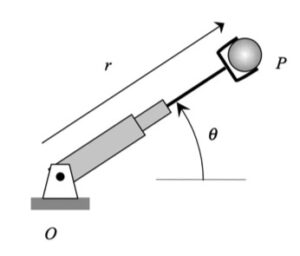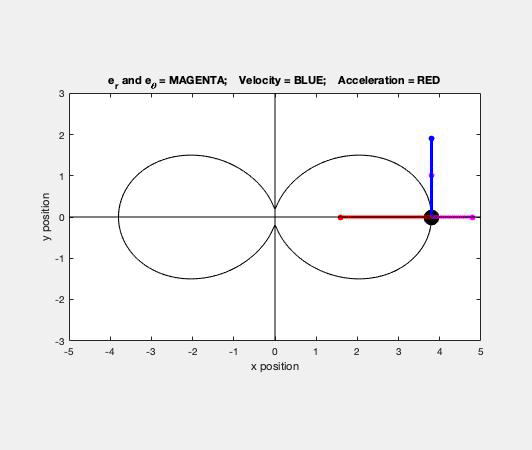# Homework H1.F - Sp23

 Problem statement Solution videoDiscusssionAs you watch the sphere move through its path, focus on a few things:

• Direction of the velocity vector. The velocity of the ball is always tangent to the path, as expected. Note that the unit tangent vector e_t points in the same direction as the velocity.
• Direction of the acceleration vector. The acceleration of the ball always points "inward" on the path, again, as expected. When P is slowing down, you see that the acceleration vector points in the negative e_t direction. Similarly, when P is increasing in speed, the acceleration vector points in the positive e_t direction.
• Directions of the polar unit vectors. The e_r unit vector always points outward from  point O toward the sphere. The e_θ unit vector is perpendicular to e_r, and in the direction of increasing θ, as seen in the animation above.

Your task is to calculate R, R_dot and R_ddot using the given equation relating R and θ. This will require derivatives with respect to TIME; however, since R is given in terms of θ, you will need to use the chain rule of differentiation. For example: R_dot = dR/dt = (dR/dθ)(dθ/dt).

## 17 thoughts on “Homework H1.F - Sp23”

1.Abraham Pugh says:

When finding dr/dtheta for r_dot, does the chain rule apply to the theta inside the cosine of the r equation. As in when I take the derivative of cosine (2 theta) and I am multiplying it by the derivative of 2 theta would that mean I am multiplying it by 2 theta_dot. So the derivative of sin(2 theta) would be cos(2 theta) * 2 * theta_dot or would it just be cos(2 theta)* 2.

1.CMK says:

THE FIRST: the derivative (with respect to time) of sin(2 theta) would be cos(2 theta) * 2 * theta_dot.

1.Abraham Pugh says:

Thank you. So just to confirm...if we had for example r_dot=sin(2*theta) and we wanted to find r_doubledot, then due to r_doubledot = dr_dot/dt = dr_dot/d theta * d theta/ dt......r_doubledot would be 2*(theta_dot squared) * cosine(2*theta).

2.Madeline B says:

With our units of rad * m / s how do we eliminate radians in order to get a m/s units for our velocity?

1.Abraham Pugh says:

I believe radians takes the form of the unit that it is multiplied with as I think it is unitless. So, in your example rad * m/s would just become m/s.

3.Luis Martinez says:

I would reccomend to look at the graph and animation for this problem and compare to your answers, and if you look closely the velocity vector never completely dissapears which I originally had thought.

1.Blake Charles Boyer says:

Are you sure that the velocity vector is never zero? Also, that might not apply to er and etheta as they are broken up with polar coordinates. It looks like one of the components will be zero at some point, just not the overall magnitude.

4.Kai Redler says:

For this problem are we determining the velocity and acceleration at a specific time/radian or just finding the equations?

1.Qianyu Zhao says:

You should plug in given values for your equations.

5.Trysta Chiang says:

When we initially plug in the given values into the r(theta) formula, do we plug in the values for R0 and R1 only or do we also plug in the value of theta?

6.CMK says:

You should always wait until the final step to plug in any numbers.

7.Zachary William Delahunty says:

In lecture we were given the derivatives of er and etheta but they aren't used in the given acceleration and velocity equations for either the single or double derivative to my understanding. Are they used in the equations at all?

1.CMK says:

I am not sure what you are asking. On page 38 of the lecture book, the derivatives of e_r and e_theta were derived. Both of these results were used in the development of the polar velocity and acceleration - without those results we would not have found those equations.

If you are asking if you will need to use the derivatives of the polar unit vectors in your calculations - probably not.

Let me know if I am missing your questions.

1.Zachary William Delahunty says:

That answered my question. Thank you for clarifying

8.Elijah Allan Collins says:

I have a quick theoretical question. Is r_dot_dot= d/dt(theta_dot*dr/d theta) the same thing as r_dot_dot = d r_dot/dt * d theta/dt

1.Elijah Allan Collins says:

Sorry-that is supposed to be r_dot_dot = d r_dot/d theta * d theta/dt

9.eaub says:

Are we supposed to plug the given value of theta into our final response? Or leave it as a function?